Next: CHOICE OF A MODEL Up: Data modeling by least Previous: FEWER EQUATIONS THAN UNKNOWNS

# HOUSEHOLDER TRANSFORMATIONS AND GOLUB'S METHOD

Our previous discussions of least squares always led us to matrices of the form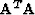which then needed to be inverted. Golub's method of using Householder transformations works directly with the matrix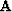and has the advantage that it is considerably more accurate than methods with invert.It seems that about twice as much precision is required to invertthan is needed to deal directly with.Another reason for learning about Golub's method is that the calculation is organized in a completely different way; therefore, it will often turn out to have other advantages or disadvantages which differ from one application to the next.

A reflection transformation is a matrix of the form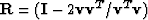where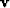is an arbitrary vector. Obviously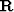is symmetric, that is,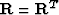.It also turns out that the reflection transformation is its own inverse, that is,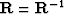.To see this, we verify by substitution that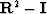.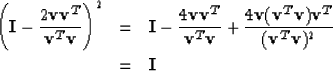(50)

A matrix transformation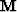is said to be unitary if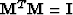.When a matrixis unitary it means that the vector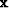has the same length as the vector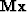.These lengths are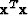and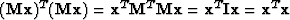which are the same. Reflection transformations are unitary because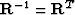.They have a simple physical interpretation. Consider an orthogonal coordinate system in which one of the coordinate axes is aligned along thevector. Reflection transformation reverses the sign of this coordinate axis vector (since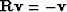) but it leaves unchanged all the other coordinate axis vectors. Thus it is obvious geometrically that reflection transformations preserve lengths and that applying the transformation twice returns any original vector to itself. Now, we seek a special reflection transformation called the Householder transformation which converts a matrix of the form on the left side to the form on the right where a is an arbitrary element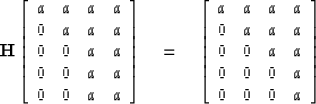(51)

Having determined the required transformation, we will know how to convert any matrix to an upper triangular form like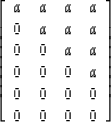(52)

by a succession of Householder transforms. Golub recognized the value of this technique in solving overdetermined sets of simultaneous equations. He noted that when the error vector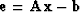is transformed by a unitary matrix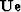the problem of minimizing the length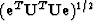ofby variation ofreduces to exactly the same problem as minimizing the length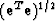ofwith respect to variation of.Thus a succession of Householder transforms could be found to reduceto the form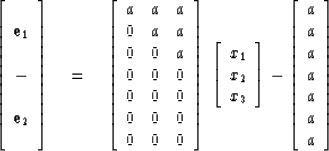(53)
Now for the clever observation that because of the zeros in the bottom part of the transformedmatrix there is no possibility of choosing any xi values which alter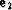in any way. The top part of the transformedmatrix is an upper triangular matrix which for any value of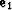can be solved exactly for the xi. The least-squares solution xi is the one for whichhas been set equal to zero.

Now we turn to the task of finding the special reflection transformation, called the Householder transformation, which accomplishes (51). Observe that the left-hand operator below is a reflection transformation for any numerical choice of s.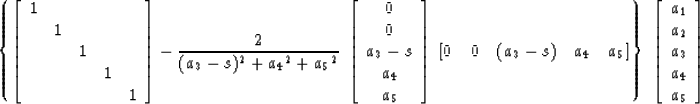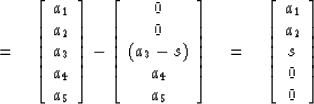(54)

Alternatively, if (54) is to be valid, then s must take a particular value such that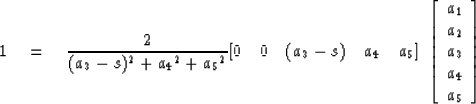(55)

or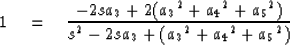(56)

This will be true only for s given by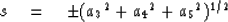(57)

Now let us see why the left-hand operator in (54) can achieve (51). Choice of the a vector as the third column in the matrix of (51) introduces the desired zeros on the right-hand side. Finally, it is necessary also to observe that this choice of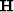does not destroy any of the zeros which already existed on the left-hand side in (51).

A subroutine for least squares fitting programmed by Don C. Riley. (This program does not do the square matrix case. It is necessary that M > N.)

        SUBROUTINE GOLUB  (A,X,B,M,N)
C
C       A(M,N) ; B(M)   GIVEN WITH M>N    SOLVES FOR X(N) SUCH THAT
C                  ||  B - AX || = MINIMUM
C       METHOD OF G.GOLUB,  NUMERISCHE MATHEMATIK  7,206-216 (1965)
C
IMPLICIT DOUBLE PRECISION (D)
REAL A(M,N),X(N),B(M),U(50)
C.........DIMENSION U(M)
C.........PERFORM N ORTHOGONAL TRANSFORMATIONS TO A(.,.) TO
C.........UPPER TRIANGULARIZE THE MATRIX
DO 3010 K=1,N
DSUM=0.0D0
DO 1010 I=K,M
DAJ=A(I,K)
1010  DSUM=DSUM+DAJ**2
DAI=A(K,K)
DSIGMA=DSIGN(DSQRT(DSUM),DAI)
DBI=DSQRT(1,0D0+DAI/DSIGMA)
DFACT=1.0D0/(DSIGMA*DBI)
U(K)=DBI
FACT-=DFACT
KPLUS=K+1
DO 1020 I=KPLUS,M
1020	U(I)=FACT*A(I,K)
C.........I - UU' IS A SYMMETRIC, ORTHOGONAL MATRIX WHICH WHEN APPLIED
C......... TO A(.,.) WILL ANNIHILATE THE ELEMENTS BELOW THE DIAGONAL K
DO 2030 J=K,N
c.........APPLY THE ORTHOGONAL TRANSFORMATION
FACT=0.0
DO 2010 I=K,M
2010  FACT=FACT+U(I)*A(I,J)
DO 2020 I=K,M
2020	A(I,J)=A(I,J)-FACT*U(I)
2030	CONTINUE
FACT=0.0
DO 2040 I=K,M
2040	FACT=FACT+U(I)*B(I)
DO 2050 I=K,M
2050	B(I)=B(I)-FACT*U(I)
3010	CONTINUE
C.........BACK SUBSTITUTE TO RECURSIVELY YIELD X(.)
X(N)=B(N)/A(N,N)
LIM=N-1
DO 4020 I=1,LIM
IROW=N-I			! error in first edition, was =N-1
SUM=0.0
DO 4010 J=1,I
4010	SUM=SUM+X(N-J+1)*A(IROW,N-J+1)
4020  X(IROW)=(B(IROW)-SUM)/A(IROW,IROW)
RETURN
END


Householder transformations can also be used in problems with constraints. In the set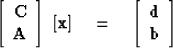(58)

one may desire to satisfy the top block exactly and the bottom block only in the least-squares sense. Define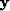as a succession of Householder transforms on;for example,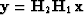.Then substitute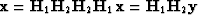into (58). Householder transforms used as postmultipliers on the matrix of (58) can be chosen to introduce zeros in the top two rows of (58), for example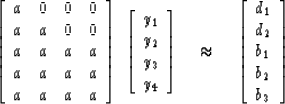(59)

Now we could use premultiplying Householder transforms on (59) to bring it to the form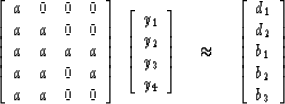(60)

Since the top two equations of (59) or of (60) are to be satisfied exactly, then y1 and y2 are uniquely determined. They cannot be adjusted to help attain minimum error in the bottom three equations. Likewise the top two equations place no restraint on y3 and y4, so they may be adjusted to produce minimum error in the bottom three equations. No amount of adjustment in y3 and y4 can change the amount of error in the last equation, so we can ignore the last equation in the determination of y3 and y4. The third and fourth equations can be satisfied with zero error by suitable choice of y3 and y4. This must be the minimum-squared-error answer. Givenwe can go back and getwith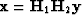.Next: CHOICE OF A MODEL Up: Data modeling by least Previous: FEWER EQUATIONS THAN UNKNOWNS
Stanford Exploration Project
10/30/1997